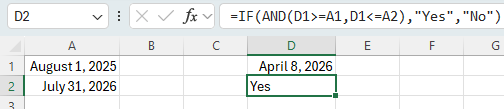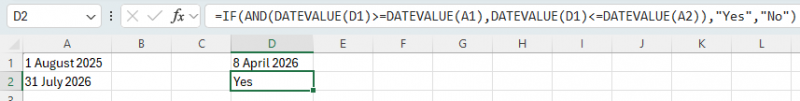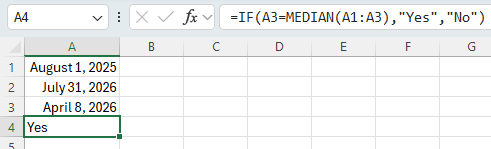# Determining If a Date is between Other DatesWritten by Allen Wyatt (last updated December 21, 2022)
This tip applies to Excel 2007, 2010, 2013, 2016, 2019, and Excel in Microsoft 365

Johna needs to determine if a particular date is between two other dates. For instance, she may need to determine if November 8, 2018, is between August 1, 2018, and July 31, 2019. She is trying to figure out the formula that will make this determination.

There are actually a wide range of formulas you could use, ranging from the short to the very long. In this tip, though, I'll focus on the shorter formulaic approaches. Before doing so, it is good to remember that dates (and times) are stored internally by Excel as numbers. The portion of the date before the decimal point (the integer portion of the number) is the serial number for the date, and the portion to the right of the decimal place is the time.

Since dates and times are stored as numbers, it becomes a relatively easy task to simply compare the numbers to determine which is higher or lower and if something is between the high and low. For instance, let's assume that you have your starting date (August 1, 2018) in cell A1 and your ending date (July 31, 2019) in cell A2. You could place the date to check into cell D1 and use the following formula:

```=IF(AND(D1>=A1,D1<=A2),"Yes","No")
```

The formula checks to see if the date in D1 is both greater than or equal to A1 and less than or equal to A2. Only if those two conditions are met is "Yes" returned, otherwise "No" is returned. (See Figure 1.)Figure 1. Comparing numbers to determine which is higher or lower and between the high and low.

If you think that the dates include times, then you may want to adjust for that by stripping off the portion of the serial number that represents the time:

```=IF(AND(TRUNC(D1,0)>=TRUNC(A1,0),TRUNC(D1,0)<=TRUNC(A2,0)),"Yes","No")
```

If you are actually comparing text strings and not recognized Excel dates, then you'll need to use the DATEVALUE function to convert the strings into dates:

```=IF(AND(DATEVALUE(D1)>=DATEVALUE(A1),DATEVALUE(D1)<=DATEVALUE(A2)),"Yes","No")
```

The result of using the DATEVALUE function would be similar to the following figure: (See Figure 2.)Figure 2. Comparing text strings using the DATEVALUE function to convert strings into dates.

Each of the formulas, so far, has been the same basic formula; the only thing different between them is the adding of additional functions to compensate for the characteristics of how the dates are stored in the cells. For an entirely different way to determine if a date is between two other dates, you could use the following formulaic approach:

```=IF(D1=MEDIAN(D1,A1,A2),"Yes","No")
```

Since the MEDIAN function is calculated using three numbers, it always returns the number that is between the lower and the higher number. This means that if D1 is really between the other two, it will always be returned; if D1 is not between the other two, then one of the others will be returned.

Interestingly, if you reorganized your data a bit so that the three values were adjacent to each other (for instance, by moving the comparison date from D1 to A3), then you could replace the three separate cell references with a range of the three cells, making the formula even shorter:

```=IF(A3=MEDIAN(A1:A3),"Yes","No")
```

The advantage to using the MEDIAN approach to the AND approach is that you don't need to worry which of the range values (A1 or A2) is the start or the end date for the comparison—the MEDIAN function sorts that all out. (See Figure 3.)Figure 3. Comparing values using the MEDIAN funtion.

ExcelTips is your source for cost-effective Microsoft Excel training. This tip (12742) applies to Microsoft Excel 2007, 2010, 2013, 2016, 2019, and Excel in Microsoft 365.

##### Author Bio

Allen Wyatt

With more than 50 non-fiction books and numerous magazine articles to his credit, Allen Wyatt is an internationally recognized author. He is president of Sharon Parq Associates, a computer and publishing services company. ...

##### MORE FROM ALLEN

Creating a Function Inventory for a Workbook

Your worksheets are very often made up of formulas and these formulas are made up of functions. If you ever want to ...

Discover More

Applying Formatting to Words

You don't have to select whole words before applying direct character formatting. With the proper Word options set, ...

Discover More

Custom Formats for Scientific Notation

Excel allows you to format your numeric values in a wide variety of ways. One such formatting option is to display ...

Discover MoreSolve Real Business Problems Master business modeling and analysis techniques with Excel and transform data into bottom-line results. This hands-on, scenario-focused guide shows you how to use the latest Excel tools to integrate data from multiple tables. Check out Microsoft Excel 2013 Data Analysis and Business Modeling today!

##### More ExcelTips (ribbon)

Date for Next Wednesday

When working with dates, it is often helpful to be able to calculate some date in the future based on a starting date. ...

Discover More

Determining If a Year is a Leap Year

Need to figure out if a given year is a leap year? It's not as easy as you think! This tip provides a plethora of ways ...

Discover More

Years in Which a Date Occurred on a Particular Day

If you need to know the years in which a particular date occurred on a specific day of the week, there are a number of ...

Discover More
##### Subscribe

FREE SERVICE: Get tips like this every week in ExcelTips, a free productivity newsletter. Enter your address and click "Subscribe."

If you would like to add an image to your comment (not an avatar, but an image to help in making the point of your comment), include the characters [{fig}] (all 7 characters, in the sequence shown) in your comment text. You’ll be prompted to upload your image when you submit the comment. Maximum image size is 6Mpixels. Images larger than 600px wide or 1000px tall will be reduced. Up to three images may be included in a comment. All images are subject to review. Commenting privileges may be curtailed if inappropriate images are posted.

What is 6 + 7?

2023-01-25 12:42:30

J. Woolley

Re. my previous comment below, My Excel Toolbox now includes the DateTimeValue function to convert date and/or time text to an Excel date serial number. For example,
=DateTimeValue("01/19/23 1:55 pm")
returns the numeric value 44945.5798611111, which can be formatted as Date (with time) or Time (with date).
Any text recognized as date and/or time will be converted. For example, "Jan 19, 23 13:55" or "19-Jan-23" or "1-19-23" or "1:55:01 pm" etc. If DateTimeValue's argument represents only a time, its result is like Excel's TIMEVALUE function. If DateTimeValue's argument represents only a date, its result is like My Excel Toolbox's DATEVALUE2 function described in my comment at https://excelribbon.tips.net/T007014

Here is an abbreviated version:

Function DateTimeValue(DateTime As Variant) As Date
DateTimeValue = CDbl(CDate(DateTime))
End Function

Notice DateTime can be text like "01/19/23 1:55 pm" or numeric like 44945.5798611111; numeric values are returned directly.

2023-01-19 14:38:13

J. Woolley

@claire
I believe my previous comment was incomplete. The Tip already explains that Excel handles time as the fractional part of a date serial number and discusses conversion of text dates into date serial numbers. I'll try again.
You might be comparing date/time values that are text like "01/19/23 1:55 pm" instead of date serial numbers. The DATEVALUE function converts a text date/time value into an integer date serial number without a fractional time part; therefore, the time part is zero (midnight). The TIMEVALUE function converts a text date/time value into the fractional time part of a date serial number; therefore, the date part is ignored. To convert a text date/time value into a date serial number that includes both the integer date part and the fractional time part, use the following cell function:
=DATEVALUE("01/19/23 1:55 pm")+TIMEVALUE("01/19/23 1:55 pm")
If that date/time text is in cell \$A\$1, use this cell function:
=DATEVALUE(\$A\$1)+TIMEVALUE(\$A\$1)
For this example, the numeric result is 44945.5798611111. Press Ctrl+1 to format a cell with a serial number like that as Date (with time) or Time (with date).
Be aware that Excel's DATEVALUE function thinks the 21st century ends after 2029, so DATEVALUE("12/07/41") returns 12/07/1941 instead of 12/07/2041. This could be a problem for the current and future decades. For more on this subject, see https://excelribbon.tips.net/T007014_Converting_Coded_Dates_into_Real_Dates.html

2023-01-18 14:48:45

J. Woolley

@claire
You might be comparing dates that are text like "01/18/23" instead of date serial numbers. Use the DATEVALUE function to convert a text date into a date serial number, then you can compare it with other date serial numbers. Press Ctrl+1 to format a cell containing the serial number as Date or Time.

2023-01-17 19:12:01

claire

How do you format a date/time when you need to find if a date/time falls between two date/times? I keep getting FALSE when I can see that the date/time is within the range.

2020-09-06 10:19:35

J. Woolley

@Matt
If I understand your question, this formula should work (assuming column B is sorted smallest to largest):
==VLOOKUP(\$A\$1,\$B:\$D,3)
The contents of column C are ignored.

2020-09-05 11:43:11

Matt

I'm trying to return a value in one column based on the week of the year and today's date. Here's how the table is set up:

A1=TODAY()
Column B is a list of dates for Monday of a given week
Column C is a list of dates for Sunday of the same week
Column D is a list of values

I would like to return the value for D1 if today (A1) is between (or equal to) B1 and C1, D2 if today (A1) is between (or equal to) B2 and C2, etc... I've tried to modify some of the formulas suggested here and come up blank.

Suggestions?

2020-09-05 10:53:22

Matt

I'm trying to return a value in one column based on the week of the year and today's date. Here's how the table is set up:

A1=TODAY()
Column B is a list of dates for Monday of a given week
Column C is a list of dates for Sunday of the same week
Column D is a list of values

I would like to return the value for D1 if today (A1) is between (or equal to) B1 and C1, D2 if today (A1) is between (or equal to) B2 and C2, etc... I've tried to modify some of the formulas suggested here and come up blank.

Suggestions?

2020-04-22 13:32:03

Fred

Harold,

I tried this and had to modify it (removed "+1" on the DAY) to get it to exept date from 1 year ago.

=IF(J3="","Inc",IF(J17="","Inc",IF(J3>J17+DATEDIF(J17,DATE(YEAR(J17)+1,MONTH(J17),DAY(J17)),"d"),"Failed","Pass")))

But it does not make a fail if someone accidently puts in a date after todays date.

Thanks for the help, but it has gone to acceptance already.

2020-04-21 12:05:25

Harold Druss

Hi Fred
A little revision. This will account for a leap year.
Adds 366 days for a non leap year, and 367 for leap year.

=IF(J3="","Inc",IF(J17="","Inc",IF(J3>J17+DATEDIF(J17,DATE(YEAR(J17)+1,MONTH(J17),DAY(J17))+1,"d"),"Failed","Pass")))

2020-04-16 21:26:27

Peter Atherton

You can make use of VBs ISDATE function as in the following

Function FindDate(ref)
Dim c
For Each c In ref
If IsDate(c) Then
Exit Function
End If
Next c
End Function

2020-04-16 07:45:33

Fred

Harold Druss,

It does work, but I had to change the "365" to "366", because it(365) doesn't include todays day exactly 1 year ago as passing.

Thanks for you help.

2020-04-15 10:26:55

Harold Druss

Hi Fred
Try this, I think it's what you want. I don't know much about Excel.
I tested for empty cells first, then added 365 days to the expiration date.

=IF(J3="","Inc",IF(J17="","Inc",IF(J3>J17+365,"Failed","Pass")))

2020-04-14 10:29:11

Fred

Allen,

I have been trying to figure this out for a while now, hope you can help.

This is a Pass/Fail for equipment that has a 1 year calibration life.

J3 = Today
J17 = Calibration date
K17 = "P+"/"<F>"/"Inc" (Pass/Fail/Incomplete

a) Formula needs to return "Pass" if J17 is equal to or between J3 and J3 - 1 year.
b) Return an "Inc" if there is no data in either J3 or J17.

This is what I have.

=IF(AND(OR(J17<=J3,J17>=(J3-366)),"P+","<F>"),OR(J17="","Inc"))

Thanks,

2020-03-18 11:56:34

Hi,

How to identify the date in the following columns.

C2 = Numbers
D2 = Blank
E2 = Blank
D2 = Date
E2 = Blank
F2 = Numbers

2020-03-15 13:45:41

Scoffers

How would you extend this function for the following adaptation.

You are checking a column of values to still see is a value falls between a start and an end date but say the start and end date relates to a policy period i.e. 1st December 2017 to 30th November 2018 and this corresponds with a policy year value of 2017. There could also be other annual periods say 1st December 2018 to 30th November 2019 with a policy year of 2018 and so on. Say these start and end dates and policy year are in a table.

The column of dates that you are checking say relate to a date of an insurance claim and you are wanting to filter this column by adding a formula in the next column to show the appropriate policy year. I'm not sure the AND function works here? Also I'm not sure a VLOOKUP works either nor INDEX and MATCH.

Any ideas?

2019-09-01 05:28:09

SteveJez

JD,
Assuming your formula as written has a typo, should have parenthesis after B2 & not comma.
Your current formula will return blank - the final argument in the formula. For your formula to work both parts of the AND function must be satisfied in order to return true to the if statement - in your example the value of C2 is returned if the logical test is true.
If there is no end date in column B then the 2nd half of the AND function is not true & therefore the AND function returns false to the IF function, resulting in the FALSE element of the IF function being returned "".

From your description is seems only the start date is important to check, therefore your formula can be reduced to ;
=IF(D1>=A2,C2,"")

I hope I have interpreted your query correctly.

2019-08-31 06:23:05

JD

Hi. Can you please help me. I already have the IF formula where i want to generate a specific value from a column if between two dates.
Example:
Column A1 Start Date, Column B1 End Date, Column C1 Headcount
Data in 2nd row: Start date Jan. 1, 2014 up to Jan.31, 2015, headcount of 7.
FOrmula in Column D2:
=IF(AND(D1>=A2, D1 <= B2, C2, "")

The formula is working fine but the problem is when there is blank or no date in End date column, no value is returned. I would like to return the value in C2 whether there is end date or not. I would like to make use of one formula only that will work whether the End date has data or not.

Your help is very much appreciated. Thanks

2019-07-21 20:19:39

Moi

Hi Allan,

I am looking to create IF function that does the following.

-If the date is less than the reference date it should mark "Not Due"
-If the date is the same or +3 days the reference date it should mark "Ok"
-If the date is more than 3 days the reference date it should mark "late"
-If it is blank or #N/A it should mark as no update

Thanks I hope you can help me with this one.

2019-05-30 09:27:22

Bob Schaettle

Here's a simple solution that doesn't use any date functions:
=IF((A1-D1)*(A2-D1)<0,"Yes","No")

2019-05-27 02:03:49

Thanks. Use of median is really something unthought of...Cheers

##### This Site

Got a version of Excel that uses the ribbon interface (Excel 2007 or later)? This site is for you! If you use an earlier version of Excel, visit our ExcelTips site focusing on the menu interface.